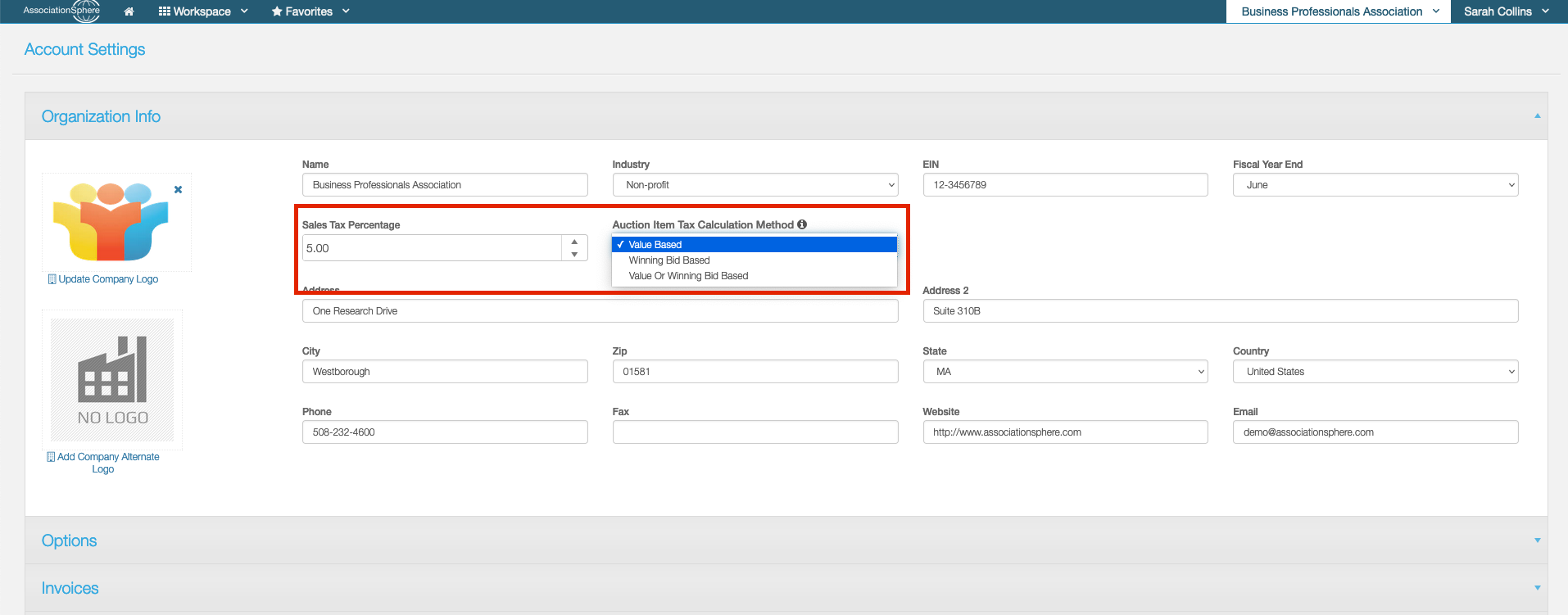For some States Sales Tax is required for any Auction Items that are sold. As each of those States have different rules regarding how the Sales Tax is calculated, we have added several options for you to choose from. The setting for Auction Item Sales Tax Calculation can be found in your Account Settings. If you are an Admin user mouse over your Organization’s Name in the upper right-hand corner of the software and click on Account.Once on the main Account screen, you will find a dropdown field titled, “Auction Item Tax Calculation Method,” which appears to the right of where you would enter in your State’s Sales Tax Percentage. Within the picklist for the “Auction Item Tax Calculation Method” dropdown, there are 3 options: Value Based, Winning Bid Based and Both. Below are specifics for each of those settings as well as examples.

Value Based - The Sales Tax on an Auction Item will be calculated based on the Item Value regardless of the Winning Bid Amount.

Winning Bid = \$50 (Less than Item Value)

Item Value = \$100

Sales Tax = 5%

Item Value (Item Value*Sales Tax) = Total

100 (100*.05) = \$105 Total Charge

Or

Winning Bid = \$500 (Greater than Item Value)

Item Value = \$100

Sales Tax = 5%

Item Value (Item Value*Sales Tax) = Total

100 (100*.05) = \$105 Total Charge

Winning Bid Based - The Sales Tax on an Auction Item will be calculated based on the Winning Bid Amount regardless of the Item Value.

Winning Bid = \$50 (Less than Item Value)

Item Value = \$100

Sales Tax = 5%

Winning Bid (Winning Bid*Sales Tax) = Total

50 (50*.05) = \$52.50 Total Charge

Or

Winning Bid = \$500 (Greater than Item Value)

Item Value = \$100

Sales Tax = 5%

Winning Bid (Winning Bid*Sales Tax) = Total

500 (500*.05) = \$525.00 Total Charge

Both - The Sales Tax on an Auction Item will be calculated based on Item Value if the Winning Bid is greater or equal than the Item Value. If the Winning Bid is less than the Item Value, then the Sales Tax will be calculated based on the Winning Bid.

Winning Bid = \$50 (Less than Item Value)

Item Value = \$100

Sales Tax = 5%

Winning Bid (Winning Bid*Sales Tax) = Total

50 (50*.05) = \$52.50 Total Charge

Or

Winning Bid = \$500 (Greater than Item Value)
Item Value = \$100

Sales Tax = 5%

Item Value (Item Value*Sales Tax) = Total

100 (100*.05) = \$105 Total Charge